# Double Factorials

As you know, the factorial of $$n$$—written $$n$$!—is the product $$n \times (n-1) \times (n-2) \times \cdots \times 1$$. The double factorial of $$n$$—written $$n!!$$—is the product $$n \times (n-2) \times (n-4) \times \cdots$$, down to 1 if $$n$$ is odd or 2 if $$n$$ is even. So $$5!! = 5 \times 3 \times 1 = 15$$, and $$6!! = 6 \times 4 \times 2 = 48$$.

I was as surprised as anyone to learn that the double factorial isn’t $$n$$ factorial factorial, $$(n!)!$$. To say nothing of the subfactorial $$!n$$.

## Stirling Permutations

Double factorials figure into the counts of Stirling permutations. Stirling permutations are permutations of $$1,1,2,2,\dots,k,k$$ where, for each $$i$$, the two copies of $$i$$ are adjacent or separated only by larger numbers. For example, the Stirling permutations of {1,1,2,2} are those where no 1 appears between the 2s. The permutations of {1,1,2,2} are:

• {1, 1, 2, 2}
• {1, 2, 1, 2}
• {1, 2, 2, 1}
• {2, 1, 1, 2}
• {2, 1, 2, 1}
• {2, 2, 1, 1}

Of these, {1,1,2,2}, {1,2,2,1}, and {2,2,1,1} meet the condition. The rest of them, {1,2,1,2}, {2,1,1,2}, and {2,1,2,1}, all have at least one 1 between the pair of 2s.

Similarly, {1,1,2,2,3,3}, {1,1,2,3,3,2}, {1,3,3,2,2,1}, are examples that meet the condition, but {3,2,2,3,1,1}, {1,2,3,1,2,3}, and {1,2,3,2,3,1} and others are not.

Stirling permutations are counted by $$(2k-1)!!$$.

One way to visualize Stirling permutations is as a simple list plot. The $$(2\times 2 - 1)!! = 3!! = 3$$ Stirling permutations of {1,1,2,2}: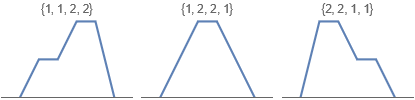The permutations that don’t meet the Stirling permutation condition have sharp peaks: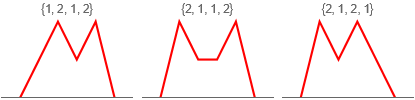The 5!! = 15 Stirling permutations of {1,1,2,2,3,3}: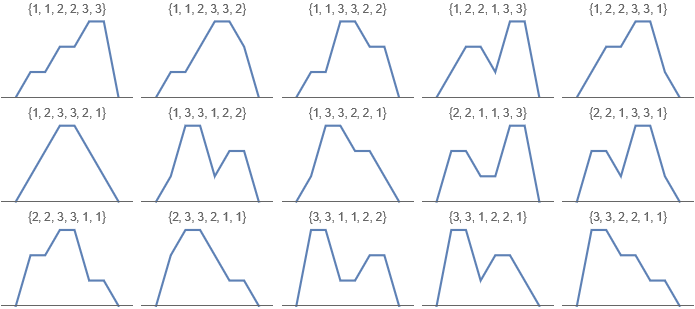The 7!! = 105 Stirling permutations of {1,1,2,2,3,3,4,4}: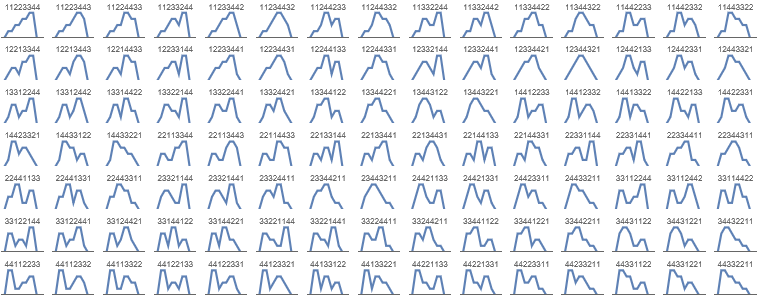And the 9!! = 945 Stirling permutations of {1,1,2,2,3,3,4,4,5,5}: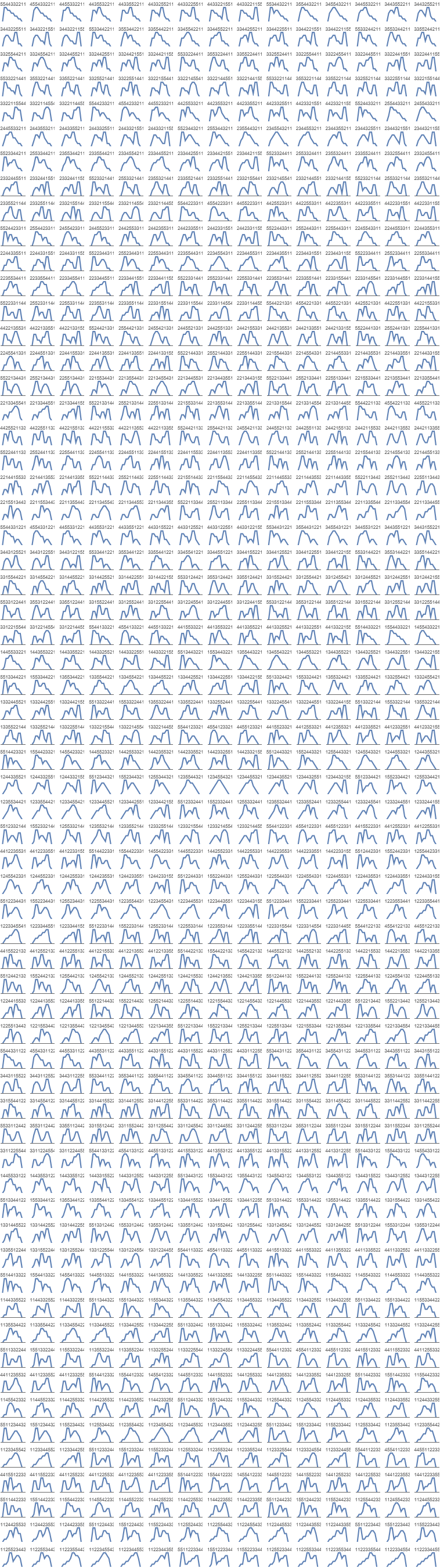See:

[ home ] || [ 2022-05-22 ]

www.robertdickau.com/doublefactorial.html